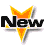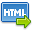Volume No. :   9

Issue No. :  3

Year :  2017

ISSN Print :  0975-4393

ISSN Online :  2349-2988Registration

## On the Complement of the Intersection Graph of Zero-Divisors of the ring Z_n

 Address: Shaik Sajana, D. Bharathi, K.K. Srimitra Department of Mathematics, S.V. University, Tirupati, A.P., India-517502. *Corresponding Author
DOI No: 10.5958/2349-2988.2017.00066.3

ABSTRACT:
For the ring of integers modulo n, we study the complement of the intersection graph of zero-divisorsis denoted by (G_Z^' (Z_n)) ¯ and is defined as a simple undirected graph whose vertices are the set of all nonzero zero-divisors of the ring Z_n and in which two distinct vertices are joined by an edge if and only if their corresponding principal ideals have zero intersection. We determine the necessary and sufficient condition for adjacency of vertices in the graph (G_Z^' (Z_n)) ¯. Also, we investigate the connectedness and further calculate the radius and diameter of the graph (G_Z^' (Z_n)) ¯ for all characterizations of n.
KEYWORDS:
Intersection graph, Zero-divisors, Principal ideal, Connected graph, Eccentricity, Radius, Diameter.
Cite:
Shaik Sajana, D. Bharathi, K.K. Srimitra. On the Complement of the Intersection Graph of Zero-Divisors of the ring Z_n. Research J. Science and Tech. 2017; 9(3):379-384.[View HTML][View PDF]

Visitor's No. :   233372